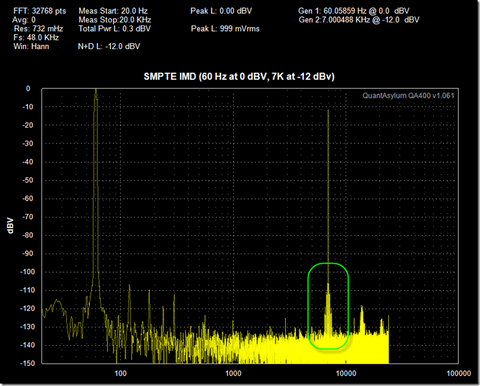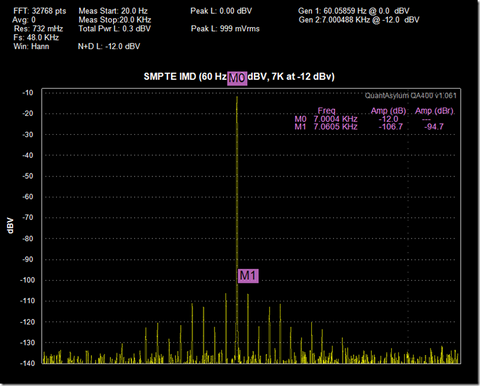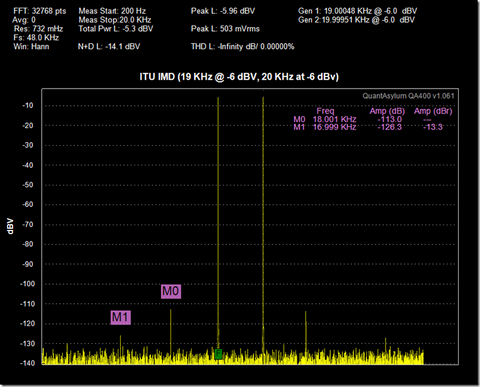# QA40x and IMD Measurements

## Intro

We will look into adding the ability to make automatic IMD (Inter-Modulation Distortion) measurements on the QA400 at some point in the future. Part of the reason we’ve held back is that there are a lot of variations that are possible in IMD measurements and it’s not yet clear if a subset will do the trick and get us 90% of the way there or not. This post will look at the types of IMD measurements, and show how these basic measurements can be made on the QA400 today.

The purpose of IMD measurements is to characterize the non-linearity of an amplifier while its being subjected to more than one tone. This is the “intermodulation” part of the Inter-Modulation Distortion Tests. THD, or Total Harmonic Distortion, is concerned with the amplifier’s ability to render a single tone.

Any amplifier that has non-linearities will “mix” two or more tones. Mixing is the process of multiplying two waveforms together. The result, in the simplest terms, is that you produce a resulting waveform that bears no sonic resemblance at all to the original tones. This is in stark contrast to harmonic distortion, where the byproducts are harmonically related to the input. In fact, in some applications (guitar fuzz), THD can even be pleasing, warm and tonally desirable. But IMD isn’t anything like this, and will most often sound completely unrelated to the source material as tones can seemingly appear out of nowhere.

And interesting experiment you can do to convince yourself of this is to use an audio program such as Audio Forge and synthesize two sine waves, one at 19 KHz and one at 20 KHz. Print them at a hot level, but not so hot that they distort. And then convert into an MP3 format at a better quality bit rate and listen to the result with headphones. What you’ll like hear is a 1 KHz tone a bit above the noise floor. Where did this 1 KHz come from? It’s the difference between the 19 KHz and 20 KHz tone: They are 1 KHz apart. Another tone was likely present at 39 KHz (the sum), but was inaudible or may have even aliased out of band.  Where did the 19 and 20 KHz tones go? They are usually too high for most people over the age of 20 to hear, but they are indeed there. And it’s not completely clear where the IMD might be happening as your amp is contributing some, but so are your headphones.

So, how can we look at IMD on the QA400?

## Popular Types of IMD Measurements

There are many types of IMD measurements to be made, but the two most popular are as follows:

SMPTE This method has been around since the late 1930’s. This test involves subjecting the device under test (DUT) to a low frequency tone (usually 60 Hz) and a mid-high tone (usually 7 KHz) and looking at the output. The ratio is also important, and the lower frequency tone is usually the stronger stimulus signal, and is usually 12 dB higher than the higher frequency tone.

ITU-R The ITU method might be even older than the SMPTE measurement, though it was originally known as the CCIF method. While the SMPTE was created by motion picture engineers, the CCIF/ITU test was created by communication engineers. In this test, two tones are generated 1 KHz apart and at the same amplitude. Usually, this is 19 KHz and 20 KHz, and then the resulting 1 KHz tone level is measured and referenced to the combined amplitude of the 19 and 20 KHz tones. But there are other variants of the test where the second and third order products are considered, or the 2nd through 5th order products are considered. The 1 KHz measurement is easy to make on the QA400.

## Measurement Plots

In setting up to make an SMPTE IMD measurement, we’ll use both generators on the QA400. Set the first generator to 60 Hz and 0 dBV, and the second generator at 7 Khz and –12 dBV (recall the SMPTE spec is that the higher frequency tone was 1/4 the level of the lower frequency tone, and 1/4th is the same as -12 dB).

In the plot below, we can see the frequency and amplitude of the tones is correct. In the green box, you can see the tone at 7 KHz has some mixing products appearing. If we turn off the 60 Hz tone, the spectrum around 7 KHz gets clear. These are indeed mixing products.If we zoom in on the 7 KHz tone, we can see the products more clearly. And indeed, measuring with markers reveals these are 60 Hz modulation products. In computing the IMD products, we’re interested in comparing the modulation products relative to the carrier, or 7 KHz tone. In the graph below, we can see a single modulation product is 94.7 dB below the 7 kHz, which means 106.7 dB below the carrier. There’s another on the other side at the same level. These two would sum and increase the overall level by 3 dB of this particular pair. So, second order IMD products would be -103.7. How far out you go generally depends on how quickly the harmonics fall off. The SMPTE spec is interested in ALL of the energy outside the 7 KHz tone, and thus it’d be correct to sum them all together. But you can get fairly accurate by simply considering the first pair or two. In this case, the 2nd order harmonics are 103.7 dB below the 60 Hz carrier, and thus the SMPTE IMD figure would be –103.7 dB or 10^(-103.7/20) * 100 = 0.000653%To make the basic ITU measurement, we set the two generators to 19 KHz at –6 dBV and 20 KHz at –6 dBV, respectively. These two tones will sum to -3 dBV, which will also be the reference stimulus for this measurement. Remember, for the ITU test, the levels will almost always be equal.Above, we can see the resulting 1 KHz mixing product. Remember, this is called a mixing product because it is the difference between the two stimulus frequencies (19 KHz and 20 KHz). There are other mixing products too. And which ones you consider are a function of the flavor you have chosen for the IMD test. In more advanced instances of this test, you might also considering the following:

1 * F1 +/- 1 * F2 = 19 +/- 20 = 39KHz and 1 KHz (2nd order IMD)

2 * F1 +/- 1 * F2 = 38 +/- 20 = 58 KHz, 18 KHz (3rd order)

1 * F1 +/- 2* F2  & 1 = 19 +/- 40 = 59 KHz and 21 KHz (3rd order)

2 * F1 +/- 2* F2 = 38 +/- 40 = 78 KHz and 2 KHz (4th order)

3 * F1 +- 2 * F2 = 57 +/- 40 = 97 KHz and 17 KHz (5th order)

2 * F1 +/- 3 * F2 = 38 +/- 60 = 98 KHz and 22 KHz (5th order)

For the simple case, we’ll consider just the 1 KHz resultant 2nd order IMD product. Here, with a 0 dB reference and a 1 KHz product at –113.4 dB, the resulting ITU IMD is –113.4 dB, or 10^(-113.4/20) * 100 = 0.000213%. To properly put this measurement in context when sharing the result, it’s very important to specify the stimulus frequencies and that only the resultant 1 KHz tone was considered.

In the plot above, we can clearly see the other frequencies around the ~20 KHz signals. Zooming in shows more detail. We can see the 18 KHz product (3rd order) and 17 KHz product (5th order). A reasonable measurement to make would be a 2nd and 3rd order measurement. In this case, we’d want to sum resulting 2nd and 3rd order harmonics and compare those to the stimulus.## Summary

A quick review was provided on the two most popular types of IMD measurements, and how to quickly make these IMD measurements on the QA400.